# | 10. + -1 points ChangWA12 6.СМ.011. My Notes Ask Your Teacher The combustion reaction of...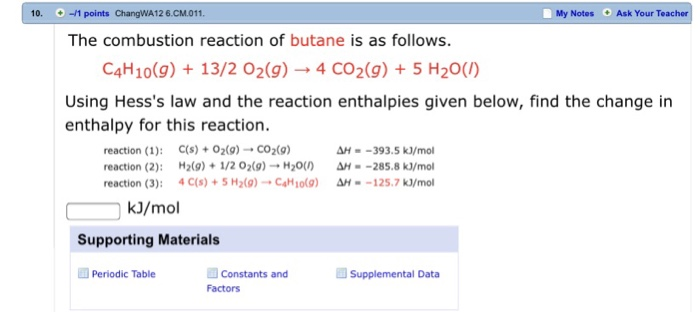| 10. + -1 points ChangWA12 6.СМ.011. My Notes Ask Your Teacher The combustion reaction of butane is as follows. C4H10(9)13/2 02(9)-4 CO2(g) 5 H20(0) Using Hess's law and the reaction enthalpies given below, find the change in enthalpy for this reaction. reaction (1): C(s) + O2(g)-. CO2(g) reaction (2): 21/2 02(9) H20UAH--285.8 kJ/mo reaction (3): 4 C(s) + 5 H2(g)- + C4H10(g) H-_1257 krmol H--393.5 kJ/mol kJ/mol Supporting Materials Periodic Table Constants and Factors Supplemental Data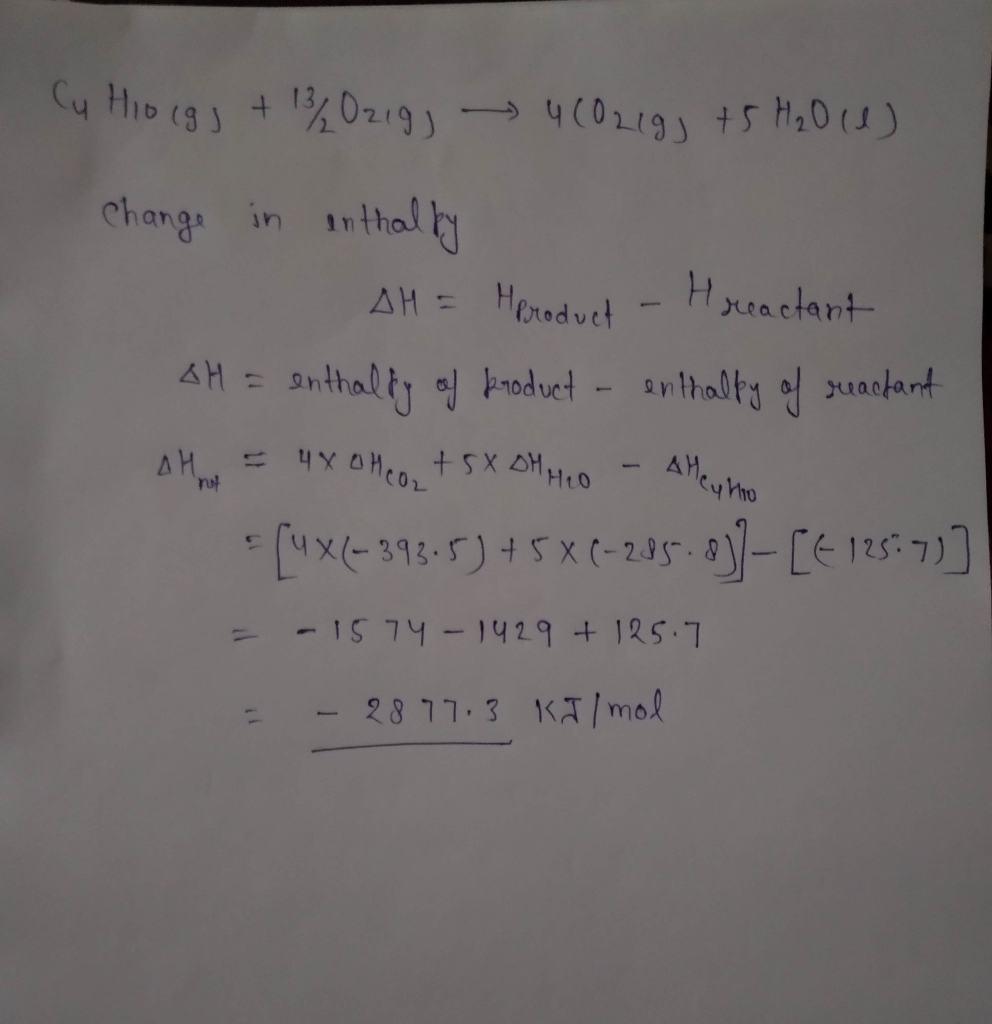Similar Homework Help Questions
• ### 16. + -/2 points was OSGenChem1 5.3.WA.033.0/10 Submissions Used The combustion reaction of propane is as...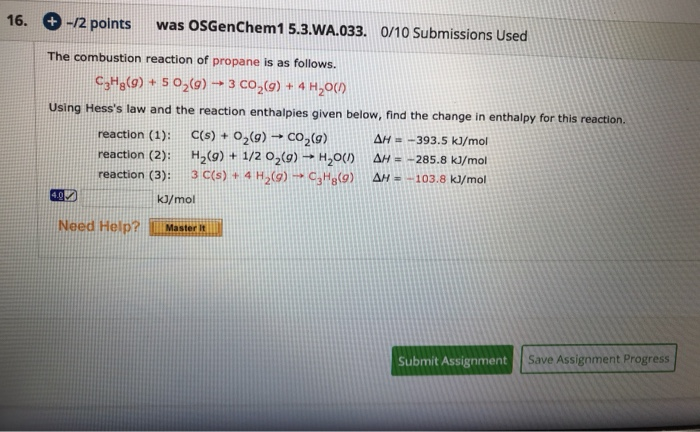16. + -/2 points was OSGenChem1 5.3.WA.033.0/10 Submissions Used The combustion reaction of propane is as follows. CHg(9) + 5 02(9) ► 3 CO2(g) + 4 H2O(1) Using Hess's law and the reaction enthalpies given below, find the change in enthalpy for this reaction. reaction (1): C(s) + O2(9) + CO2(g) | AH = -393.5 kJ/mol reaction (2): H2(9) + 1/2 02(g) → H200 AH = -285.8 kJ/mol reaction (3): 3 C(s) + 4 H (9) CHg(9) AH = -103.8...

• ### Calculate the AH formation of octane, CBH1B (), given that the enthalpy of combustion of octane...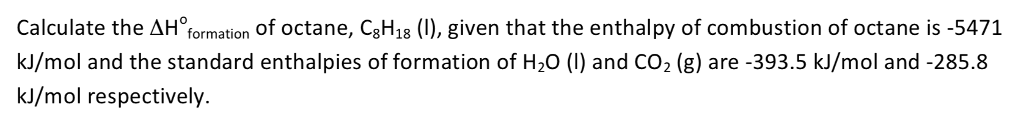Calculate the AH formation of octane, CBH1B (), given that the enthalpy of combustion of octane is -5471 kl/mol and the standard enthalpies of formation of H20 () and CO2 (g) are -393.5 kJ/mol and -285.8 kJ/mol respectively.

• ### can you do the 7 and 9 ? can you also show and explain me step...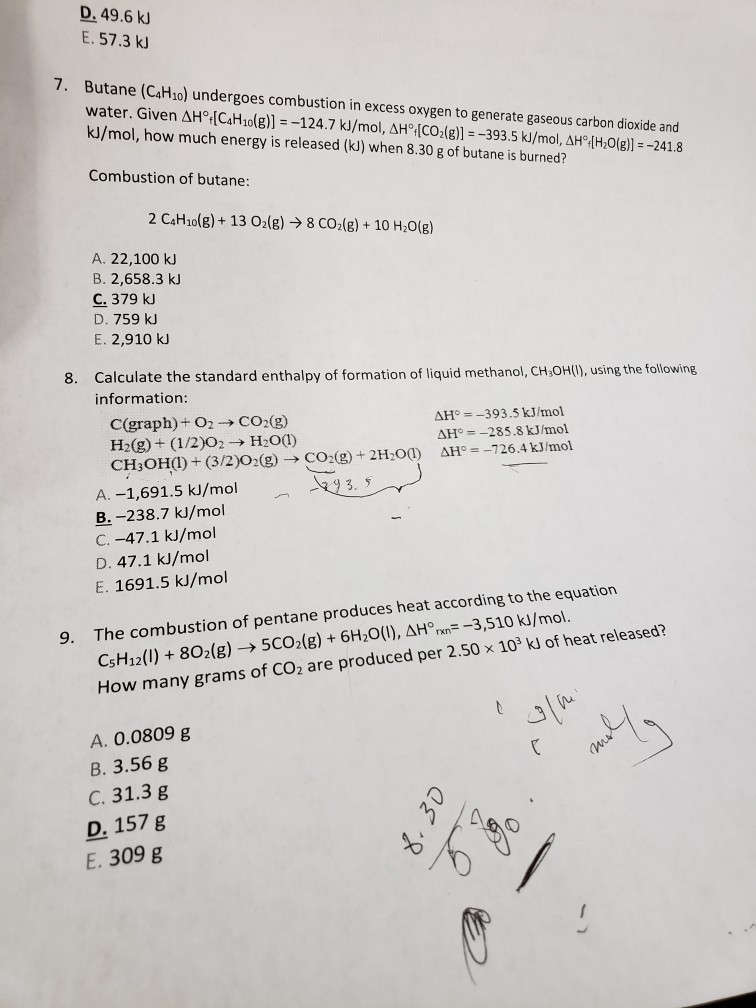can you do the 7 and 9 ? can you also show and explain me step by step? D. 49.6 kJ E. 57.3 kJ 7. Butane (C4H10) undergoes combustion in excess oxygen to generate gaseous carbon dioxide and water. Given AH° [C4H10(g)] = -124.7 kJ/mol, AH° [CO2(g)] = -393.5 kJ/mol, AH [H2O(g)] = -241.8 kJ/mol, how much energy is released (kJ) when 8.30 g of butane is burned? Combustion of butane: 2 CH2(g) + 13 O2(g) → 8 CO2(g) +...

• ### Question 20 5 pts The thermochemical equation for the combustion of butane is ---- 2C4H10(g) +...Question 20 5 pts The thermochemical equation for the combustion of butane is ---- 2C4H10(g) + 1302(g) k) 8CO2(g) + 10H2O(g) AH = -5314.6 Using the value of AH given for this reaction, and the standard enthalpies of formation (AH-values) for O2(g), CO2(g), and H2O(g), calculate the standard enthalpy of formation (AH!) for C4H10. Note the following standard enthalpies of formation: ΔΗ, OkJ/mol Substance O2(g) CO2(g) H2O(g) -393.5 kJ/mol -241.8 kJ/mol -251.4 kJ/mol 251.4 kJ/mol 125.7 kJ/mol -317.7 kJ/mol -125.7...

• ### PROBLEM-SOLVING CLASS ACTIVITY 11 Use Hess's Law to calculate the enthalpy of formation of CH2OH: C(graphite)...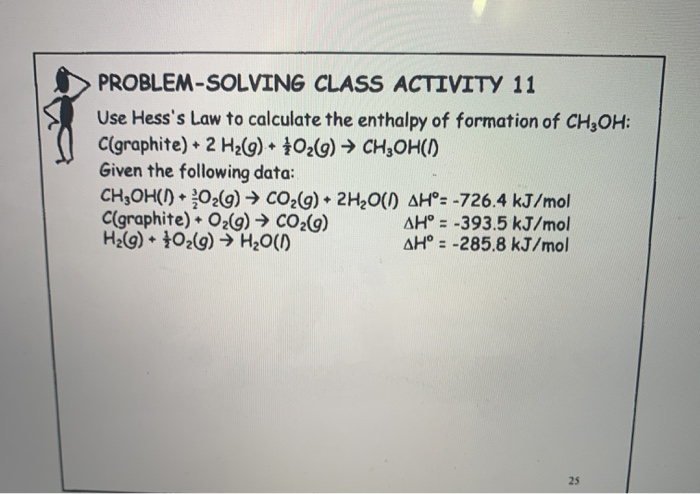PROBLEM-SOLVING CLASS ACTIVITY 11 Use Hess's Law to calculate the enthalpy of formation of CH2OH: C(graphite) + 2 H2(g) + 1026) → CH2OH(1) Given the following data: CH2OH() • 02(9) + CO2(g) + 2H2O(1) AH°: -726.4 kJ/mol C(graphite). O2(g) → CO2(9) AH' = -393.5 kJ/mol H2(g) + 40269) → H2O(1) AH = -285.8 kJ/mol

• ### Please show all steps: Calculate the enthalpy for this reaction: Given the following thermochemical equations: c:H2(g)...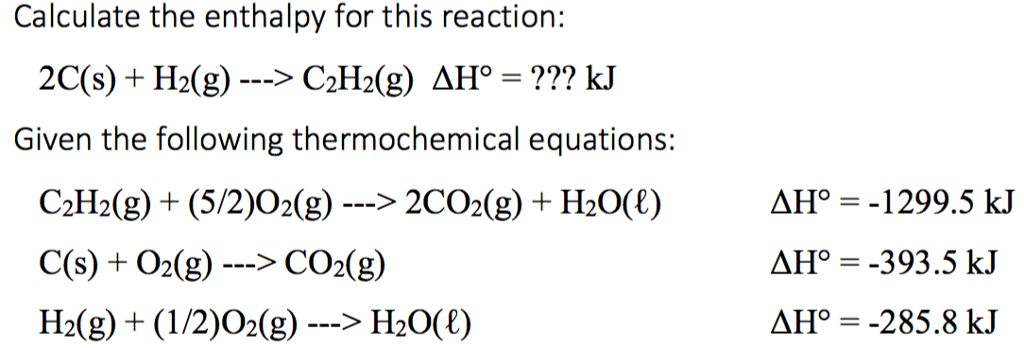Please show all steps: Calculate the enthalpy for this reaction: Given the following thermochemical equations: c:H2(g) + (5/2)?2(g) > 2CO2(g) + H20(1) C(s) +02(g) -> CO2(g) ??? =-1299.5 kJ ??? -393.5 kJ ??? 285.8 kJ -> H2

• ### Calculate the enthalpy, AHrxn for this reaction: 2 C(s) +H2 (9) ► C2H5 (9) Given: C2H5(9)...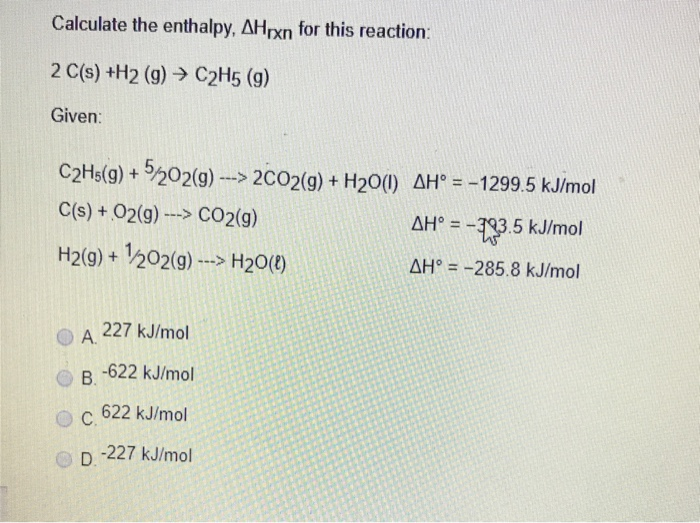Calculate the enthalpy, AHrxn for this reaction: 2 C(s) +H2 (9) ► C2H5 (9) Given: C2H5(9) + 5/202(9) ---> 2CO2(g) + H20(1) AH° = - 1299.5 kJ/mol C(s) + O2(9) ---> CO2(g) AH° = -23.5 kJ/mol H2(g) + 1202(9) ---> H20() AH' = -285.8 kJ/mol A 227 kJ/mol В. -622 kJ/mol 622 kJ/mol D -227 kJ/mol Calculate the enthalpy, AHrxn for this reaction: 2 C(s) +H2 (9) > C2H5 (9) Given: C2H5(9) + 5/202(9) ---> 2C02(g) + H20(1) AH° =...

• ###  The enthalpy of reaction for  enthalpy of reaction for the combustion of C to CO, is-393.5 kJ/mol C, and th...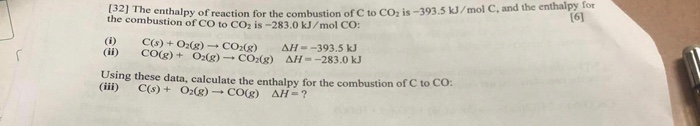The enthalpy of reaction for  enthalpy of reaction for the combustion of C to CO, is-393.5 kJ/mol C, and the enthalpy for the combustion of CO to CO, is-283.0 kJ/mol CO: (i) C(s) + O2(g) + CO2(e) AH -393.5 kJ CO(g) + O2(g) + CO2(g) AH -283.0 kJ Using these data, calculate the enthalpy for the combustion of C to CO. (iii) C(s) + O2(g) - CO(g) AH-?

• ### 6. Hess's Law. Use the data in Table 1 to calculate AH for the reaction below:...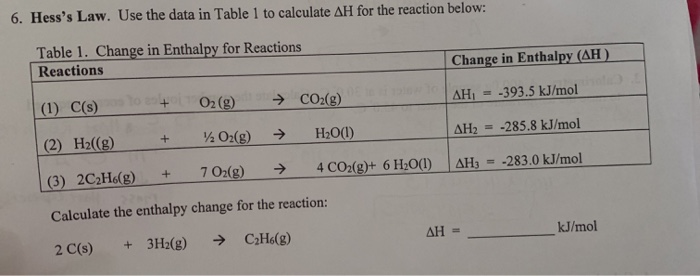6. Hess's Law. Use the data in Table 1 to calculate AH for the reaction below: Table 1. Change in Enthalpy for Reactions Reactions Change in Enthalpy (AH) AH = -393.5 kJ/mol (1) C() (2) Hz((g) + + O2(g) O2(g) → CO2(8) → H2O(l) AH2 = -285.8 kJ/mol (3) 2CH.(g) + 702(g) → 4 CO2(g)+ 6H2O(1) AH = -283.0 kJ/mol Calculate the enthalpy change for the reaction: 2 C(s) + 3H2(g) → CzH6(g) AH = kJ/mol

• ### 6. Hess's Law. Use the data in Table 1 to calculate AH for the reaction below:...6. Hess's Law. Use the data in Table 1 to calculate AH for the reaction below: Table 1. Change in Enthalpy for Reactions Reactions Change in Enthalpy (AH) AH = -393.5 kJ/mol (1) C() (2) Hz((g) + + O2(g) O2(g) → CO2(8) → H2O(l) AH2 = -285.8 kJ/mol (3) 2CH.(g) + 702(g) → 4 CO2(g)+ 6H2O(1) AH = -283.0 kJ/mol Calculate the enthalpy change for the reaction: 2 C(s) + 3H2(g) → CzH6(g) AH = kJ/mol

Free Homework App